# NCERT Exemplar solutions for Class 11 Physics chapter 7 - System of Particles and Rotational Motion [Latest edition]

#### Chapters## Solutions for Chapter 7: System of Particles and Rotational Motion

Below listed, you can find solutions for Chapter 7 of CBSE NCERT Exemplar for Class 11 Physics.

Exercises
Exercises [Pages 50 - 56]

### NCERT Exemplar solutions for Class 11 Physics Chapter 7 System of Particles and Rotational Motion Exercises [Pages 50 - 56]

#### MCQ I

Exercises | Q 7.1 | Page 50

For which of the following does the centre of mass lie outside the body?

• A pencil

• A shotput

• A dice

• A bangle

Exercises | Q 7.2 | Page 50

Which of the following points is the likely position of the centre of mass of the system shown in figure?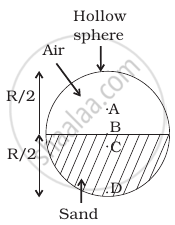• A

• B

• C

• D

Exercises | Q 7.3 | Page 50

A particle of mass m is moving in yz-plane with a uniform velocity v with its trajectory running parallel to + ve y-axis and intersecting z-axis at z = a (Figure). The change in its angular momentum about the origin as it bounces elastically from a wall at y = constant is ______.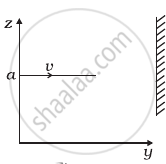• mva hate_x

• 2mva hate_x

• ymv hate_x

• 2ymv hate_x

Exercises | Q 7.4 | Page 51

When a disc rotates with uniform angular velocity, which of the following is not true?

• The sense of rotation remains same.

• The orientation of the axis of rotation remains same.

• The speed of rotation is non-zero and remains same.

• The angular acceleration is non-zero and remains same.

Exercises | Q 7.5 | Page 51

A uniform square plate has a small piece Q of an irregular shape removed and glued to the centre of the plate leaving a hole behind (Figure). The moment of inertia about the z-axis is then ______.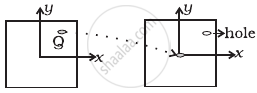• increased

• decreased

• the same

• changed in unpredicted manner

Exercises | Q 7.6 | Page 51

A uniform square plate has a small piece Q of an irregular shape removed and glued to the centre of the plate leaving a hole behind figure. The CM of the plate is now in the following quadrant of x-y plane ______.

• I

• II

• III

• IV

Exercises | Q 7.7 | Page 51

The density of a non-uniform rod of length 1 m is given by ρ(x) = a(1 + bx2) where a and b are constants and 0 ≤ x ≤ 1. The centre of mass of the rod will be at ______.

• (3(2 + b))/(4(3 + b))

• (4(2 + b))/(3(3 + b))

• (3(3 + b))/(4(2 + b))

• (4(3 + b))/(3(2 + b))

Exercises | Q 7.8 | Page 52

A Merry-go-round, made of a ring-like platform of radius R and mass M, is revolving with angular speed ω. A person of mass M is standing on it. At one instant, the person jumps off the round, radially away from the centre of the round (as seen from the round). The speed of the round afterwards is ______.

• ω

• ω/2

• 0

#### MCQ II

Exercises | Q 7.9 | Page 52

Choose the correct alternatives:

1. For a general rotational motion, angular momentum L and angular velocity ω need not be parallel.
2. For a rotational motion about a fixed axis, angular momentum L and angular velocity ω are always parallel.
3. For a general translational motion , momentum p and velocity v are always parallel.
4. For a general translational motion, acceleration a and velocity v are always parallel.
Exercises | Q 7.10 | Page 52

Figure shows two identical particles 1 and 2, each of mass m, moving in opposite directions with same speed v along parallel lines. At a particular instant, r1 and r2 are their respective position vectors drawn from point A which is in the plane of the parallel lines. Choose the correct options: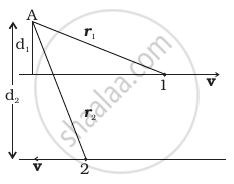1. Angular momentum l1 of particle 1 about A is l1 = mvd1
2. Angular momentum l2 of particle 2 about A is l2 = mvr2
3. Total angular momentum of the system about A is l = mv(r1 + r2)
4. Total angular momentum of the system about A is l = mv (d2 − d1)

⊗ represents a unit vector coming out of the page.

⊗ represents a unit vector going into the page.

Exercises | Q 7.11 | Page 52

The net external torque on a system of particles about an axis is zero. Which of the following are compatible with it?

1. The forces may be acting radially from a point on the axis.
2. The forces may be acting on the axis of rotation.
3. The forces may be acting parallel to the axis of rotation.
4. The torque caused by some forces may be equal and opposite to that caused by other forces.
Exercises | Q 7.12 | Page 53

Figure shows a lamina in x-y plane. Two axes z and z ′ pass perpendicular to its plane. A force F acts in the plane of lamina at point P as shown. Which of the following are true? (The point P is closer to z′-axis than the z-axis.)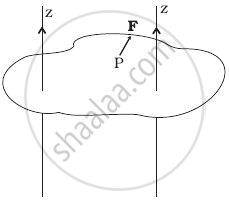1. Torque τ caused by F about z axis is along -hatk.
2. Torque τ′ caused by F about z′ axis is along -hatk.
3. Torque τ caused by F about z axis is greater in magnitude than that about z axis.
4. Total torque is given be τ = τ + τ′.
Exercises | Q 7.13 | Page 52

With reference to figure of a cube of edge a and mass m, state whether the following are true or false. (O is the centre of the cube.)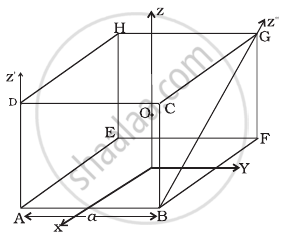1. The moment of inertia of cube about z-axis is Iz = Ix + Iy
2. The moment of inertia of cube about z ′ is I'z = I_z + (ma^2)/2
3. The moment of inertia of cube about z″ is = I_z + (ma^2)/2
4. Ix = Iy

#### VSA

Exercises | Q 7.14 | Page 53

The centre of gravity of a body on the earth coincides with its centre of mass for a ‘small’ object whereas for an ‘extended’ object it may not. What is the qualitative meaning of ‘small’ and ‘extended’ in this regard? For which of the following the two coincides? A building, a pond, a lake, a mountain?

Exercises | Q 7.15 | Page 53

Why does a solid sphere have smaller moment of inertia than a hollow cylinder of same mass and radius, about an axis passing through their axes of symmetry?

Exercises | Q 7.16 | Page 53

The variation of angular position θ, of a point on a rotating rigid body, with time t is shown in figure. Is the body rotating clock-wise or anti-clockwise?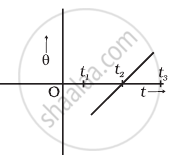Exercises | Q 7.17 | Page 54

A uniform cube of mass m and side a is placed on a frictionless horizontal surface. A vertical force F is applied to the edge as shown in figure. Match the following (most appropriate choice):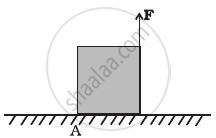(a) mg/4 < F < mg/2 (i) Cube will move up. (b) F > mg/2 (ii) Cube will not exhibit motion. (c) F > mg (iii) Cube will begin to rotate and slip at A. (d) F = mg/4 (iv) Normal reaction effectively at a/3 from A, no motion.
Exercises | Q 7.18 | Page 54

A uniform sphere of mass m and radius R is placed on a rough horizontal surface (Figure). The sphere is struck horizontally at a height h from the floor. Match the following: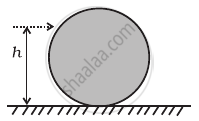Column I Column II (a) h = R/2 (i) Sphere rolls without slipping with a constant velocity and no loss of energy. (b) h = R (ii) Sphere spins clockwise, loses energy by friction. (c) h = 3R/2 (iii) Sphere spins anti-clockwise, loses energy by friction. (d) h = 7R/5 (iv) Sphere has only a translational motion, looses energy by friction.

#### SA

Exercises | Q 7.19 | Page 54

The vector sum of a system of non-collinear forces acting on a rigid body is given to be non-zero. If the vector sum of all the torques due to the system of forces about a certain point is found to be zero, does this mean that it is necessarily zero about any arbitrary point?

Exercises | Q 7.20 | Page 54

A wheel in uniform motion about an axis passing through its centre and perpendicular to its plane is considered to be in mechanical (translational plus rotational) equilibrium because no net external force or torque is required to sustain its motion. However, the particles that constitute the wheel do experience a centripetal acceleration directed towards the centre. How do you reconcile this fact with the wheel being in equilibrium? How would you set a half-wheel into uniform motion about an axis passing through the centre of mass of the wheel and perpendicular to its plane? Will you require external forces to sustain the motion?

Exercises | Q 7.21 | Page 54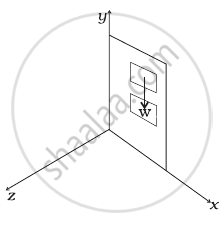Exercises | Q 7.22 | Page 55

(n – 1) equal point masses each of mass m are placed at the vertices of a regular n-polygon. The vacant vertex has a position vector a with respect to the centre of the polygon. Find the position vector of centre of mass.

Exercises | Q 7.23 | Page 55

Find the centre of mass of a uniform (a) half-disc, (b) quarter-disc.

Exercises | Q 7.24 | Page 55

Two discs of moments of inertia I1 and I2 about their respective axes (normal to the disc and passing through the centre), and rotating with angular speed ω2 and ω2 are brought into contact face to face with their axes of rotation coincident.

1. Does the law of conservation of angular momentum apply to the situation? why?
2. Find the angular speed of the two-disc system.
3. Calculate the loss in kinetic energy of the system in the process.
4. Account for this loss.
Exercises | Q 7.25 | Page 55

A disc of radius R is rotating with an angular speed ωo about a horizontal axis. It is placed on a horizontal table. The coefficient of kinetic friction is µk.

1. What was the velocity of its centre of mass before being brought in contact with the table?
2. What happens to the linear velocity of a point on its rim when placed in contact with the table?
3. What happens to the linear speed of the centre of mass when disc is placed in contact with the table?
4. Which force is responsible for the effects in (b) and (c).
5. What condition should be satisfied for rolling to begin?
6. Calculate the time taken for the rolling to begin.
Exercises | Q 7.26 | Page 55

Two cylindrical hollow drums of radii R and 2R, and of a common height h, are rotating with angular velocities ω(anti-clockwise) and ω(clockwise), respectively. Their axes, fixed are parallel and in a horizontal plane separated by (3R + δ). They are now brought in contact (δ → 0).

1. Show the frictional forces just after contact.
2. Identify forces and torques external to the system just after contact.
3. What would be the ratio of final angular velocities when friction ceases?
Exercises | Q 7.27 | Page 56

A uniform square plate S (side c) and a uniform rectangular plate R (sides b, a) have identical areas and masses (Figure).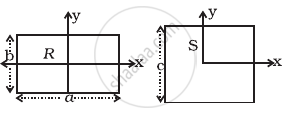Show that

1. IxR/IxS < 1
2. IyR/IyS > 1
3. IzR/IzS > 1
Exercises | Q 7.28 | Page 56

A uniform disc of radius R, is resting on a table on its rim.The coefficient of friction between disc and table is µ (Figure). Now the disc is pulled with a force F as shown in the figure. What is the maximum value of F for which the disc rolls without slipping?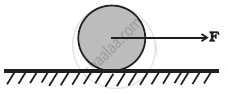## Solutions for Chapter 7: System of Particles and Rotational Motion

Exercises## NCERT Exemplar solutions for Class 11 Physics chapter 7 - System of Particles and Rotational Motion

Shaalaa.com has the CBSE Mathematics Class 11 Physics CBSE solutions in a manner that help students grasp basic concepts better and faster. The detailed, step-by-step solutions will help you understand the concepts better and clarify any confusion. NCERT Exemplar solutions for Mathematics Class 11 Physics CBSE 7 (System of Particles and Rotational Motion) include all questions with answers and detailed explanations. This will clear students' doubts about questions and improve their application skills while preparing for board exams.

Further, we at Shaalaa.com provide such solutions so students can prepare for written exams. NCERT Exemplar textbook solutions can be a core help for self-study and provide excellent self-help guidance for students.

Concepts covered in Class 11 Physics chapter 7 System of Particles and Rotational Motion are Dynamics of Rotational Motion About a Fixed Axis, Angular Momentum in Case of Rotation About a Fixed Axis, Rolling Motion, Momentum Conservation and Centre of Mass Motion, Centre of Mass of a Rigid Body, Centre of Mass of a Uniform Rod, Rigid Body Rotation, Equilibrium of Rigid Body, Moment of Inertia, Theorems of Perpendicular and Parallel Axes, Kinematics of Rotational Motion About a Fixed Axis, Motion - Rigid Body, Motion of Centre of Mass, Linear Momentum of a System of Particles, Vector Product of Two Vectors, Angular Velocity and Its Relation with Linear Velocity, Torque and Angular Momentum, Centre of Mass, Equations of Rotational Motion, Comparison of Linear and Rotational Motions, Values of Moments of Inertia for Simple Geometrical Objects (No Derivation).

Using NCERT Exemplar Class 11 Physics solutions System of Particles and Rotational Motion exercise by students is an easy way to prepare for the exams, as they involve solutions arranged chapter-wise and also page-wise. The questions involved in NCERT Exemplar Solutions are essential questions that can be asked in the final exam. Maximum CBSE Class 11 Physics students prefer NCERT Exemplar Textbook Solutions to score more in exams.

Get the free view of Chapter 7, System of Particles and Rotational Motion Class 11 Physics additional questions for Mathematics Class 11 Physics CBSE, and you can use Shaalaa.com to keep it handy for your exam preparation.